﻿ Chapter 3: Index Buffer Objects and Primitive Types | OpenGLBook.com

# Chapter 3: Index Buffer Objects and Primitive Types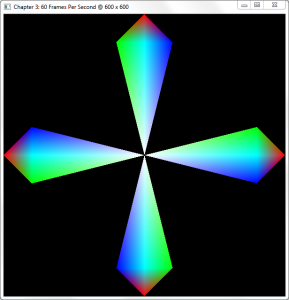Now that we’ve drawn our first geometric shapes in Chapter 2, it’s time to step up the complexity a bit. While uploading our vertices to the GPU and rendering them all as a batch is a great solution for a single triangle, you will soon notice that as geometric complexity increases, so does the need for more efficient rendering methods.

In this chapter, you’ll learn how to:

• Use index buffers (AKA Index Buffer Objects, or IBOs).
• Draw complex geometry easier.
• Use several more primitive types.

Please note that this chapter builds on the samples provided in Chapter 2, so if you haven't read it, I suggest you read it now

Check the requirements before continuing

OpenGL 3.x / DirectX 10 Level Hardware

This chapter is 100% compatible with OpenGL 3.x level hardware by changing only a few lines of code.

# The Problem

Let's say we wanted to draw the following shape onto the screen, each of the spoke’s vertices (marked by Pn) a different color: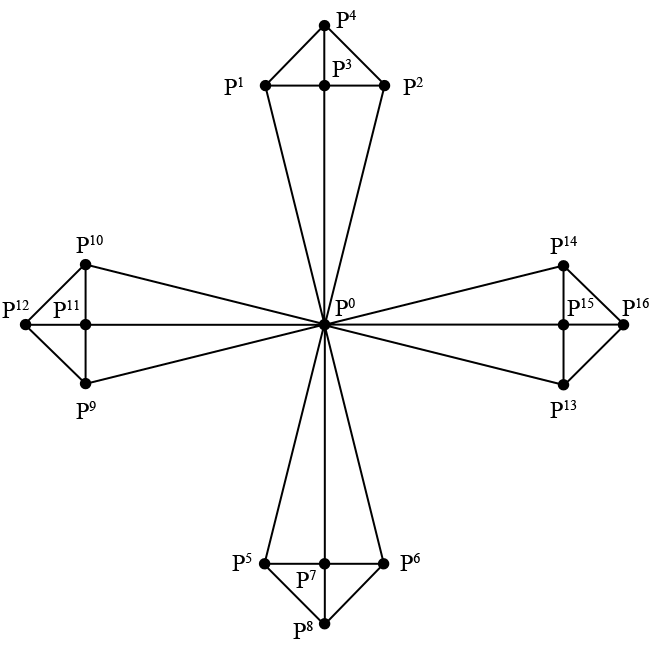## Using GL_TRIANGLES

If we were to use the previous chapter’s code, we’d have to draw out 16 individual triangles composed of 48 vertices in total using the GL_TRIANGLES primitive type since we describe each triangle separately. This means that each set of three vertices in your array describes a single independent triangle. You can see an example of this in file chapter.3.0.1.c in the download section at the end of this chapter.

In this example, P0 (origin) is duplicated 8 times in total, the center vertices P3, P7, P11, and P15 are duplicated 4 times each. If this seems like overkill to you for such a simple shape, you’re right; there are better ways of describing it.

Amount of data sent to GPU memory using GL_TRIANGLES:
(size of Vertex) 32 bytes x 48 = 1,536 bytes.

## Using GL_TRIANGLE_STRIP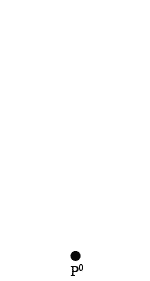The GL_TRIANGLE_STRIP primitive mode is a bit better when it comes to sheer number of vertices sent to the GPU, since we only send 28 in total. However, there’s still unneeded duplication, and the way in which we traverse the vertices is a bit cumbersome due to the way that GL_TRIANGLE_STRIP works.

The GL_TRIANGLE_STRIP primitive type creates triangles out of every newly added vertex and its preceding two vertices. In the animation, P0 to P1 doesn’t yield a triangle, but after we add P3, a triangle forms. Each vertex after the addition of P3 yields a new triangle.

In addition to the unneeded duplication (notice P3 → P4 → P3) and the amount of data sent over, there’s also no way of changing the order in which we draw the vertices besides uploading a completely new batch of data.

Amount of data sent to GPU memory using GL_TRIANGLE_STRIP:
(size of Vertex) 32 bytes x 28 = 896 bytes.

# Enter Index Buffers

So far, we’ve been drawing our geometry with a call to glDrawArrays, which simply draws a certain subset of elements from the currently active vertex buffer object. Let’s explore a new way of drawing by walking through some new code. Make a copy of chapter.2.4.c from the previous chapter and name it chapter.3.1.c.

Since we’re in chapter two of the book, change the WINDOW_TITLE_PREFIX pre-processor definition to reflect Chapter 3.

Next, change CurrentWidth to have an initial value of 600:

400: Invalid request


Add a new identifier named IndexBufferId to our list of global identifier variable declarations:

400: Invalid request


Remove the call to glDrawArrays in RenderFunction and replace it with the following line:

400: Invalid request


Here’s the big change, change the Vertices variable in CreateVBO to the following:

400: Invalid request


Immediately beneath Vertices, add the following array definition:

400: Invalid request


At the end of the CreateVBO function, right below the last call to glEnableVertexAttribArray, add the following lines of code:

400: Invalid request


Finally, add the following lines of code between the glDeleteBuffers and glBindVertexArray lines of the DestroyVBO function:

400: Invalid request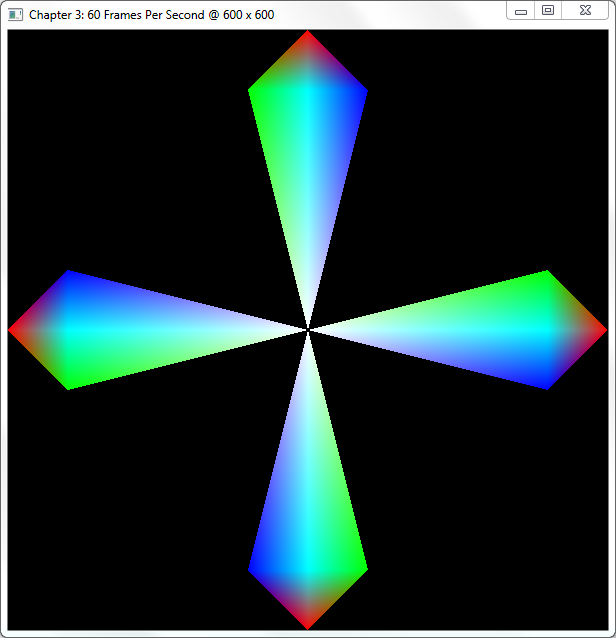## Step-By-Step

If you’ve analyzed the code a bit, you’ll notice that we upload each vertex exactly once to the GPU instead of 48 vertices including duplicates. Let’s walk through the code and take a good look at what we did here.

The first changes were purely aesthetic: as always, we changed the window title to reflect this chapter and made the window a perfect square for the geometry to show up symmetrical. In the next chapter, we’ll introduce a method that doesn’t require you to reshape your window to achieve symmetry.

The next thing we add is the IndexBufferId variable to the global list of variables. This variable will hold the buffer’s identifier generated by a call to glGenBuffers, similar to vertex buffer generation in the previous chapter.

### Modifying Vertices and Adding Indices

After that, we modify the CreateVBO function and replace its Vertices array with an array of 17 total vertices. If we were to use glDrawArrays on this VBO without indices, we would not output the desired shape as illustrated earlier. Instead, we have to define one more array, named Indices, to hold the indices of the elements in Vertices in drawing order.

For example, the first three indices 0, 1, and 3, correspond directly with the first, fourth, and second elements in the Vertices array, composing the left-bottom triangle of the shape’s top spoke. In total, we upload 48 indices since each spoke consists of four triangles (3 x 4 x 4).

Indices is defined as a GLubyte array of 48 elements; GLubyte is the OpenGL data type for an unsigned byte (unsigned char). You could use any of the following unsigned integral OpenGL data types: GLubyte, GLushort, and GLuint, since indices are never negative (signed) or fractional (float/double).

### Index Buffer Generation, Binding, and Filling

The final thing inside of the CreateVBO function is to generate the actual index buffer with a familiar call to the glGenBuffers, nothing new there. It isn’t until the next function call to glBindBuffers that we specify a brand new target that we haven’t used before.

Until now, we’ve only supplied the GL_ARRAY_BUFFER target to glBindBuffer to specify that the buffer is an array of vertices. While we still upload our vertices in this manner, our newly generated buffer is bound to the GL_ELEMENT_ARRAY_BUFFER target that allows us to specify which vertices in the active GL_ARRAY_BUFFER we’re using.

The call to glBufferData should also look very similar to last chapter’s code; we introduce no new options here besides the usage of the GL_ELEMENT_ARRAY_BUFFER target flag.

### Rendering the Indexed Vertex Buffer Object

In the code, we removed the call to glDrawArrays and replaced it with a call to a function called glDrawElements. Whereas glDrawArrays only draws the active GL_ARRAY_BUFFER, glDrawElements draws the indices of the active GL_ARRAY_BUFFER as specified by the buffer bound to the GL_ELEMENT_ARRAY_BUFFER target. Let’s take a closer look at the glDrawElements function:

400: Invalid request


The first parameter, mode, takes in the primitive mode to use such as GL_TRIANGLES or GL_TRIANGLE_STRIP; the same as the mode parameter of glDrawArrays.

The second parameter, count, specifies how many elements in total to draw. In our case, this value is 48 since that’s the amount of indices in the Indices array.

The third parameter, type, specifies which data type was used for the index array. In our case, this is GL_UNSIGNED_BYTE, since we used the GLubyte data type to construct the Indices array. Please note that this type must reflect the data type used to construct the array containing the indices.

The fourth and last parameter, indices, specifies the offset in bytes in the index array of where we want to start rendering, allowing us to render subsets of the vertex data. For example, if we wished to draw just the right-hand spoke of the shape, we’d call the glDrawElements function with the following parameters:

400: Invalid request


In this function call, we draw 12 vertices starting at index offset 36 of the index buffer, corresponding to the vertices in the Indices array. Make sure to change the parameters in glDrawElements according to the type of index buffer you're using. For example, if we were to use an index buffer containing unsigned integers (GLuint), we'd have to change the above function call to the following:

400: Invalid request


Amount of data sent to GPU memory using Index Buffers:
(size of Vertex) 32 bytes x 17 + 1 GLubyte x 48 = 592 bytes.

# Swapping Index Buffers

Sometimes it is not possible to avoid having to change the indices you wish to render, in which case it is possible to swap the active index buffer by simply changing the buffer bound to the GL_ELEMENT_ARRAY_BUFFER target.

Make a copy of chapter.3.1.c rename it to chapter.3.2.c and open it up in your editor. The first thing we do is change the block of global GLuint variable definitions to look like this:

400: Invalid request


Next, add the following function declaration right underneath the IdleFunction function declaration:

400: Invalid request


In the InitWindow function definition, add the following line underneath the call to glutCloseFunc:

400: Invalid request


Add the following function definition right below the InitWindow function definition:

400: Invalid request


Next, we need to make some changes to the rendering, RenderFunction, to facilitate the changes we've made. Replace the call to glDrawElements with the following code:

400: Invalid request


Inside of the CreateVBO function right underneath the Indices array definition, place the following code:

400: Invalid request


Inside of the same function, change the call to generate the index buffers to the following:

400: Invalid request


Immediately after that, change the glBindBuffer function call to the following:

400: Invalid request


Right before the call to glGetError, add the following block of code:

400: Invalid request


Finally, in the DestroyVBO function, change the glDeleteBuffers call with IndexBufferId as its parameter to the following:

400: Invalid request


When you run the program and press the "T" key, you are able to toggle back and forth between the original shape and this new shape:## Step-By-Step

With only a few minor changes, we were able to draw an entirely new shape out of he same set of vertices. The only thing we did to achieve this was swap the index buffers. If you examined the code, you’ll notice that we’ve covered all of the functionality used in this sample before, so we’ll just glance over some of the highlights.

In this sample, we changed the amount of index buffers generated to two to contain an alternate index buffer for our swapping purposes. In the CreateVBO function, we updated glGenBuffers to generate two buffer objects and store their identifiers in the array IndexBufferId, which now contains two elements.

After that, we upload the new indices stored in AlternateIndices to the GPU’s memory using glBufferData as usual and set the current active index buffer back to the original.

We also added some new FreeGLUT functionality for handling keyboard input:

400: Invalid request


The Key parameter contains the character representation of the key pressed, while the X and Y parameters contain the mouse positions relative to the window at the time of the key-press. The only thing we do in this function is toggle back and forth between index buffers while retaining the same vertex data.

We register the call to this function in the InitWindow function with a call to glutKeyBoardFunc, which takes in as its only parameter a function pointer, just as any other FreeGLUT callback functions do.

# More Primitive Types

Until now, we’ve only discussed GL_TRIANGLES and GL_TRIANGLE_STRIP as methods to describe geometry. However, there are several more so-called "primitive types" available in OpenGL, each of which alters the output in a different way. In this section, we’ll discuss a few more.

Primitives

A "primitive" is the smallest component of a geometrical shape. So far, we’ve used triangles as our primitive types but OpenGL supports two others: points and line segments.

## GL_POINTS

The first and simplest primitive type is GL_POINTS, where each vertex specifies a visible point in space. When using GL_POINTS, OpenGL will draw simple points onto the screen. For example, change the glDrawElements in chapter.3.1.c to use GL_POINTS instead of GL_TRIANGLES, and each vertex will show up as a colored one-pixel point.

You can change the point-size with the function glPointSize, which simply takes in a single parameter, size, specifying the size of the points as a floating-point number.

## GLLINESTRIP

The second primitive type is GL_LINE_STRIP, which allows us to draw lines between vertices. GL_LINE_STRIP works much like GL_TRIANGLE_STRIP, where each new vertex adds to the overall line instead of defining a brand new line every two vertices.

To try this primitive type, add one more index to 0 at the very end of the index array named Indices in chapter.3.1.c, and change the call to glDrawElements to the following:

400: Invalid request


You should now see the outline of the shape described at the beginning of the chapter.

## GL_LINE_LOOP

The third primitive type, GL_LINE_LOOP is very similar to GL_LINE_STRIP with the exception that it closes the line segment by drawing a line between the last vertex and the first. Whereas we added one more index to Indices for GL_LINE_STRIP, we would not have to do this with GL_LINE_LOOP.

## GL_LINES

GL_LINES is to lines what GL_TRIANGLES is to triangles, meaning that GL_LINES describes separate, unconnected lines. However, since a line consists of two points instead of the three required by a triangle, changing the sample in chapter.3.1.c does not yield a desired result. We would have to modify the index array extensively in order to get the correct results.

## GL_TRIANGLE_FAN

The last primitive type to discuss in this section is GL_TRIANGLE_FAN, a close relative to the well-known GL_TRIANGLE_STRIP. Whereas GL_TRIANGLE_STRIP constructs a new triangle by connecting the last three points added to the list, GL_TRIANGLE_FAN constructs a new triangle from the very first point and the last two points added, resulting in a fan-like shape. This means that every new triangle is connected to the very first added to the list.

There is no correct way of drawing the shapes presented in this chapter using GL_TRIANGLE_FAN, but this primitive type is useful for drawing center-oriented polygons, such as a pentagon with all of its vertices connecting in the center.

## Polygon Rasterization Modes

If you’re looking to display your geometry as a wireframe of its original, there’s no need to change its primitive type. The function glPolygonMode can take care of this by changing the method used to fill the triangles, a process called “rasterization” that we’ll explore in a future chapter:

400: Invalid request


The function’s first parameter, face, specifies which polygons of your geometry the function affects. As of OpenGL 3.0, this parameter may only be set to GL_FRONT_AND_BACK; we’ll learn more about front- and back-faces in a future chapter.

The function’s second parameter, mode, specifies how the polygons are rasterized. This mode can be set to one of the following values:

• GL_POINT Draws the geometry as points, related to the GL_POINTS primitive type.
• GL_LINE Draws the geometry as lines (wireframe), related to the GL_LINES primitive type.
• GL_FILL The default: draws each triangle filled with a solid color.

Below follows a combination of three screenshots showing all of the different rasterization modes for the example shown earlier in this chapter: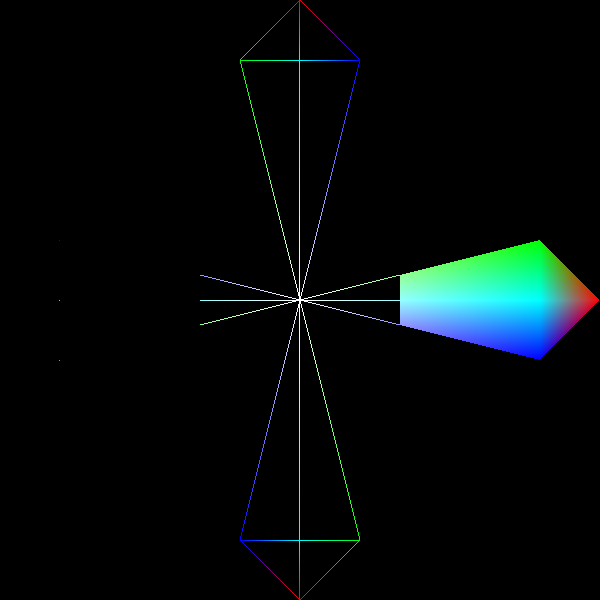# Conclusion

Index Buffer Objects can be incredibly useful when dealing with complex shapes by limiting the amount of data sent to the GPU. The fact that many model formats provide their data in separate vertex and index sections only makes the decision to use index buffers more natural.

In upcoming chapters, index buffers are the primary method to describe geometry, so try to get familiar with this chapter and modify the samples to draw some geometry of your own. In the next chapter, we're drawing our first three-dimensional geometry.

You can find the source code for the samples in this chapter here.